### Home > CC4 > Chapter 7 > Lesson 7.1.4 > Problem7-49

7-49.
1.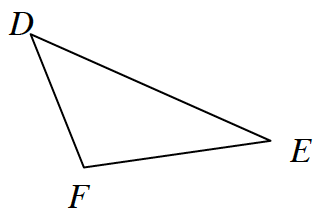Examine the triangle at right. Each part is a separate problem. Homework Help ✎

1. If mD = 48º and mF = 117º, then what is mE?

2. Solve for x if mD = 4x + 2º, mF = 7x – 8º, and mE = 4x + 6º. Then calculate mD.

3. If mD = mF = mE, what type of triangle is ΔFED?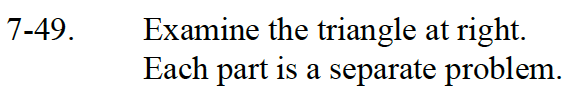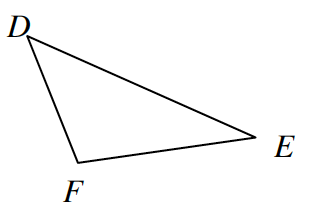Review the Math Notes Box in this Lesson 7.1.4.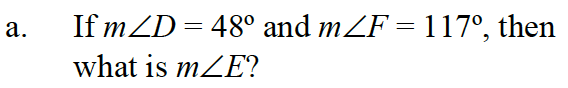Use the Triangle Angle Sum Theorem: 48° + 117° + mE = 180°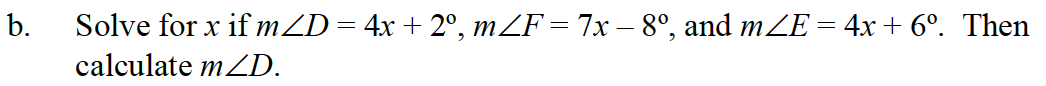Use the same method as part (a).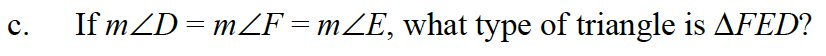If all of the angles have equal measure, then all of the sides have equal measure.